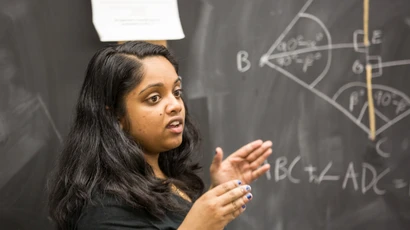# Get Started in the Department of Mathematical Sciences

## Where Should a Student Begin in Math?

MATH 122 – University Calculus I (CCC – Mathematics/Quantitative Reasoning)
Placement: The student should have passed 4 years of college-preparatory mathematics in high school, with a B or better in the 4th-year course and an 85 or better on the NYS Algebra 2 and Trigonometry (or Course B) Regents, or should have passed MATH 106 (or equivalent).
Intended audience: Students majoring in mathematics, science, cooperative engineering or computer science.  This is also an excellent course for students with a good mathematics background and an interest in mathematics.

MATH 120 – Survey of Calculus I (CCC – Mathematics/Quantitative Reasoning)
Placement: The student should have passed 4 years of college-preparatory mathematics in high school, with an 85 or better on the NYS Algebra 2 and Trigonometry (or Course B) Regents, or should have passed MATH 105 with a C+ or better.
Intended audience: Students majoring in business administration, social science, biology, or computer science.  This course covers much of the same material as MATH 122, but not in as much depth. Also, there is more emphasis on business applications than in MATH 122.  Note that students who take this course and later decide to switch to a major requiring MATH 122 will need to take an additional course.  Good mathematics students should take MATH 122.
Credit will not be given for both MATH 120 and MATH 122.

MATH 110 – Mathematics in Action (CCC – Mathematics/Quantitative Reasoning)
Placement: The student should have passed the NYS Algebra 2 and Trigonometry (or Course B) Regents.
Intended audience: This course was designed for the College Core Curriculum. It is an excellent course for students in the arts or humanities who wish to explore some useful quantitative concepts but do not plan to take additional mathematics courses.  For majors in Early Childhood or Childhood Education, MATH 110 (or MATH 117) satisfies one of the requirements for the Mathematics Concentration.  The course examines topics not generally covered in high school, such as design of street networks, planning and scheduling, voting systems, and measuring populations.

MATH 108 – Prize-Winning Mathematics (CCC – Mathematics/Quantitative Reasoning)
Placement: The student should have a 75 or better on the NYS Algebra 2 and Trigonometry (or Course B) Regents.
Intended audience: Students majoring in business administration or social science.  Topics will be selected from systems of equations, matrix algebra, inequalities and linear programming, probability, games and decisions, and Markov chains.  This course includes applications to business, economics, and the mathematics of finance.

STAT 150 – Statistical Ideas (CCC – Mathematics/Quantitative Reasoning)
Placement: The student should have passed the NYS Algebra 2 and Trigonometry (or Course B) Regents.
Intended audience: The purpose of this course is to introduce students to the field of statistics, to examine the role that it plays in our lives, and to help students avoid being hoodwinked by the misuse of statistics.  This course was designed for the College Core Curriculum.  The emphasis is on data and concepts rather than on calculations and mathematical theory.

STAT 200 – Statistical Methods (CCC – Mathematics/Quantitative Reasoning)
Placement: The student should have passed the NYS Algebra 2 and Trigonometry (or Course B) Regents.
Intended audience: This course is designed to introduce students to the use of statistical techniques in business, economics, education, and the social sciences.

STAT 250 - Statistics for Scientists (CCC – Mathematics/Quantitative Reasoning)
Placement: The student should have passed the NYS Algebra 2 and Trigonometry (or Course B) Regents.
Intended Audience: This course is designed for students majoring in Biology, Chemistry, Geosciences or Physics and emphasizes uses of statistics in the natural sciences. Topics include descriptive statistics, data collection, probability distributions, confidence intervals, hypothesis testing, regression, and analysis of variance.  The course includes use of analytical labs and statistical computer packages.

MATH 117 – Why Math? (CCC – Natural Sciences)
Placement: The student should have passed the NYS Algebra 2 and Trigonometry (or Course B) Regents.
Intended audience: For students who wish to learn about the nature of mathematics and what mathematicians do.  For majors in Early Childhood or Childhood Education, MATH 117 (or MATH 110) counts toward the Mathematics Concentration.  There is an emphasis in the course on concepts and ideas, and their historical development, rather than on calculations.

MATH 106 – University Precalculus (This course does not fulfill a CCC requirement.)
Placement: The student should have a 75 or better on the NYS Algebra 2 and Trigonometry (or Course B) Regents.
Intended audience: For students who need additional preparation for calculus. Specifically, this course is designed to prepare students to take MATH 122 – University Calculus I.  It will review algebra, and cover solving inequalities, algebraic and transcendental functions, trigonometry, analytic geometry, applications, and computational technology.
Note: MATH 106 is not a CCC course.

MATH 105 – Survey of Precalculus (This course does not fulfill a CCC requirement.)
Placement: The student should have passed the NYS Algebra 2 and Trigonometry Course (or Course B).
Intended audience: For students who plan to take MATH 120 – Survey of Calculus I, but need additional preparation; in particular, for those who took the NYS Algebra 2 and Trigonometry course (or Course B) but scored less than 75 on the Regents exam.  This course will review algebra and geometry, and cover algebraic, exponential, and logarithmic functions, transformations and combinations of functions, solving inequalities and systems of equations, and computational technology.
Note: MATH 105 is not a CCC course.

Questions?  e-mail: math@fredonia.edu   or call: 716-673-3243